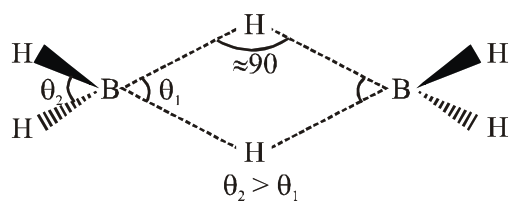`
Question:

The correct statement about $\mathrm{B}_{2} \mathrm{H}_{6}$ is:

1. Terminal B-H bonds have less p-character when compared to bridging bonds.

2. The two B-H-B bonds are not of same length

3. All B-H-B angles are of $120^{\circ}$

4. Its fragment, $\mathrm{BH}_{3}$, behaves as a Lewis base

Correct Option: 1

Solution:- $\quad \theta_{2}>\theta_{1}, \therefore \mathrm{B}-\mathrm{H}$ (terminal) having less $\mathrm{p}-$ character as compare to bridge bond.

- Both B-H-B bridge bond having same bond length.

- B-H-B bond angle is $\approx 90^{\circ}$

- $\quad \mathrm{BH}_{3}$ is $\mathrm{e}^{-}$deficient species and therefore act as lewis acid